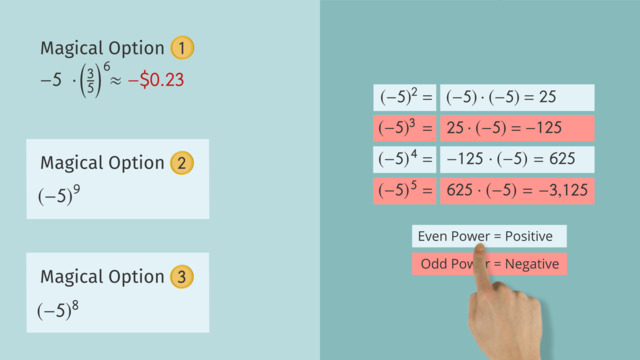# Exponential NotationRating

Ø 5.0 / 1 ratings
The authorsChris S.

## Basics on the topicExponential Notation

After this lesson you will be able to use exponents, and determine whether a number raised to a given power will be positive or negative.

The lesson begins with the definition of exponential notation. This leads to an explanation of why negative numbers raised to an even power produce positive results, while negative numbers raised to an odd power produce negative results.

Learn about exponents by helping Wade and Skeeter decide what to do with the magical fish!

This video includes key concepts, notation, and vocabulary such as: base (the number being multiplied); exponent (the number of times the base is being multiplied by itself); the phrase, “x raised to the nth power” (where n is the exponent and x is the base); square (x raised to the second power is called the “square” of x); and cube (x raised to the third power is called the “cube” of x).

Before watching this video, you should already be familiar with exponential notation using base 10, and multiplication as repeated addition.

After watching this video, you will be prepared to learn about fractional or negative exponents.

Common Core Standard(s) in focus: 8.EE.A.1 A video intended for math students in the 8th grade Recommended for students who are 13-14 years old

## Exponential Notation exercise

Would you like to apply the knowledge you’ve learned? You can review and practice it with the tasks for the video Exponential Notation.
• ### Identify the key aspects of exponential notation.

Hints

Exponential Notation is a shortcut used to express repeated multiplication.

$5^2 = 5\cdot5$

$5^3 = 5\cdot5\cdot5$

$5^4 = 5\cdot5\cdot5\cdot5$

$3^5$ is written in exponential notation.

We can read this as "three to the fifth power".

To evaluate this expression, we can multiply 3 by itself, five times.

$3\cdot3\cdot3\cdot3\cdot3 = 243$

Solution

This expression is written in exponential notation.

It is read as "six to the fourth power".

The $6$ is the base and the $4$ is the exponent.

The expression expanded would be $\mathbf{6\cdot6\cdot6\cdot6}$.

The solution is $\mathbf{1,296}$.

• ### Expanding expressions written in exponential notation.

Hints

Remember, when the negative sign is inside the parentheses, the number is negative and is repeatedly multiplied.

$(-n)^3=(-n)(-n)(-n)$

If the negative sign is outside the parentheses, the number is positive which is being repeatedly multiplied, and the negative sign is applied at the end.

$-(w)^3=-(w)(w)(w)$

Solution

The solution is $\mathbf{(-7)(-7)(-7)(-7)}$.

Since the negative sign is inside the parentheses with the 7, it is a negative 7 that will multiplied by itself four times.

• ### Identify each expression in exponential notation.

Hints

Pay attention to the differences between the two numbers used for exponential notation. The larger number is the base, and the smaller number is the exponent.

For example: $5^3$

The $5$ is the base, and the $3$ is the exponent.

The base is multiplied by itself the amount of times the exponent says.

$(-x)^5=(-x)(-x)(-x)(-x)(-x)$

Solution

$(-3)^4=(-3)(-3)(-3)(-3)$

$4^3 = (4)(4)(4)$

$3^4 = (3)(3)(3)(3)$

$3^3=3\cdot3\cdot3$

$(-4)^4=(-4)(-4)(-4)(-4)$

• ### Identify the sign of each expression in exponential notation.

Hints

When the base is negative, it is important to pay attention to the exponent to see if it is even or odd.

Even Exponent = Positive Result

Odd Exponent = Negative Result

The base can be any negative value for this rule to apply.

For example:

$(-m)^\mathbf{2}$ The exponent of $2$ is even, so this equals a positive answer.

$(-m)^\mathbf{3}$ The exponent of $3$ is odd, so this equals a negative answer.

Solution

$(-h)^{2}$ = positive

$(-3)^{18}$ = positive

$(-4)^{6}$ = positive

$(-5)^{8}$ = positive

$(-m)^{10}$ = positive

$(-1)^{3}$ = negative

$(-e)^{1}$ = negative

$(-9)^{7}$ = negative

$(-2)^{15}$ = negative

$(-w)^{11}$ = negative

• ### Rewrite the expression using exponential notation.

Hints

Here, the $3$ is the base and the $4$ is the exponent. As you can see, the $3$ will be multiplied by itself $4$ times in order to find the solution.

This can be true for any number written in exponential notation.

The base is the number we multiply by itself. And the exponent tells us how many times.

For the expression $2\cdot2\cdot2\cdot2$, the $2$ is the base, since that is the number that is being multiplied repeatedly.

Solution

The base is 2 and the exponent is 4.

$2^4$

• ### Interpret values in exponential notation.

Hints

Remember, the location of the negative sign can change the solution to an expression in exponential notation. If you are unsure whether the final solution will be a positive or negative value, you can always follow these steps:

1. Expand the expression to show repeated multiplication.
2. Follow the order of operations to find the solution.

Don't forget the rules for multiplying integers!

When the signs are the same, the product is positive.

When the signs are different, the product is negative.

$(-3)(-3)$

This has two negative numbers, so our product must be a positive nine ($9$).

$(-3)(3)$

One number is negative, and the other is positive, so the product must be a negative nine ($-9$).

See if this can help you with a better understanding of which expressions equal 9 and which equal $-9$.

Solution

The following expressions are equal to $9$:

$(3^2)$

$(-3)^2$

$3^2$

$(-3)(-3)$

$9^2$

$(3)(3)$

The following expressions are equal to $-9$.

$-9^1$

$-(3)^2$

$(-9)^1$

$-(3^2)$

$-(3)(3)$

$-3^2$